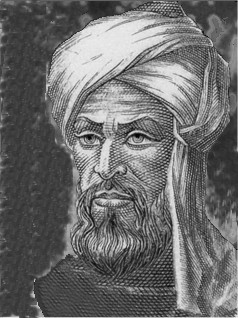# Algebra Seminar

• M 4:00-5:00pm
• UH 4170

## Next Talk

• December 4, 2017
• Mihai D. Staic, (BGSU)
• Secondary Hochschild Cohomology
• Abstract: In this talk I will give an introduction to secondary Hochschild Cohomology, discussing the initial motivation and the main properties for this construction.

## Talks This Semester

• December 4, 2017
• Mihai D. Staic, (BGSU)
• Secondary Hochschild Cohomology
• Abstract: In this talk I will give an introduction to secondary Hochschild Cohomology, discussing the initial motivation and the main properties for this construction.

• November 27, 2017
• Alimjon Eshmatov
• On quivers and their representations II
• Abstract: We continue our discusson of Gabriel's theorem and related things.

• November 6, 2017
• Alimjon Eshmatov
• On quivers and their representations
• Abstract: A quiver is a directed graph. A representation of a quiver is an assignment of a vector space to each vertex and a linear map to each arrow. One of the most fundamental theorems in this theory is Gabriel's theorem. In this talk I will state this theorem and outline its proof.

• October 23, 2017
• Nate Iverson
• Quadratic forms over finite fields
• Abstract: Will define quadratic form and prove the equivalency of the 2 rival definitions for any field. Discuss non-degeneracy (in arbitrary characteristic), equivalence of forms, and classify all non-degenerate quadratic forms over a finite field (up to change of variable). We will explicitly cover the characteristic 2 case and mention the Chevalley-Warning theorem and Witt's theorem (for extending isometries).

• September 25, 2017
• Alimjon Eshmatov
• Noncommutative deformation of Kleinian singularities
• Abstract: Associated to a finite subgroup $G$ of $SL_2 (\mathbb{C})$, there is a family of noncommutative algebras representing a deformation of the coordinate ring of the classical Kleinian singularity $\mathbb{C}^2//G$. These algebras found to be related to many areas of mathematics: mathematical physics, algebraic geometry, symplectic geometry to name few. In the talk, we will give overview of these algebras and related results.